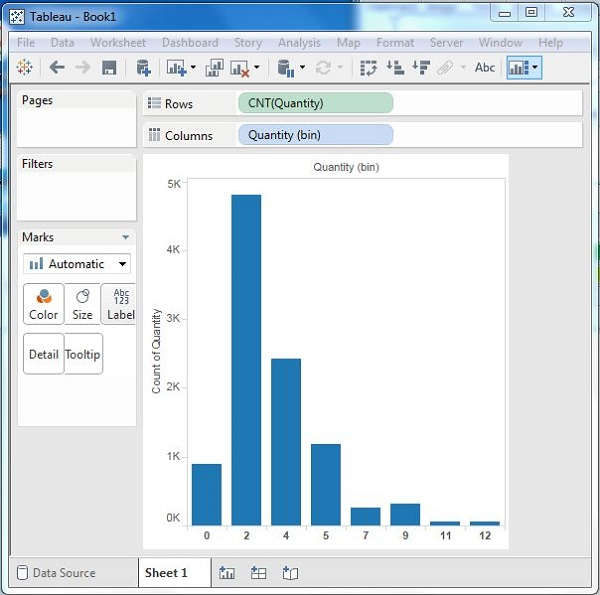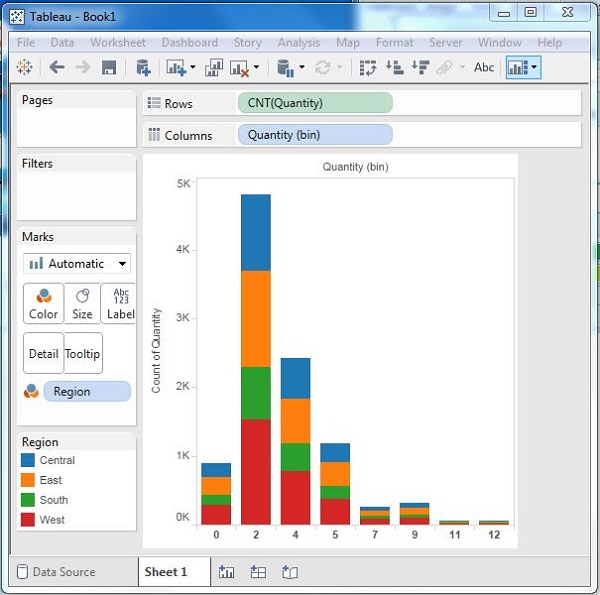# Tableau - Histogram

A histogram represents the frequencies of values of a variable bucketed into ranges. Histogram is similar to bar chart but it groups the values into continuous ranges. Each bar in histogram represents the height of the number of values present in that range.

Tableau creates a histogram by taking one measure. It creates an additional bin field for the measure used in creating a histogram.

## Creating a Histogram

Using the Sample-superstore, plan to find the quantities of sales for different regions. To achieve this, drag the Measure named Quantity to the Rows shelf. Then open Show Me and select the Histogram chart. The following diagram shows the chart created. It shows the quantities automatically bucketed into values ranging from 0 to 4811 and divided into 12 bins.### Creating a Histogram with Dimension

You can also add Dimensions to Measures to create histograms. This will create a stacked histogram. Each bar will have stacks representing the values of the dimension. Following the steps of the above example, add the Region Dimension to the color Shelf under Marks Card. This creates the following histogram where each bar also includes the visualization for different regions.